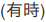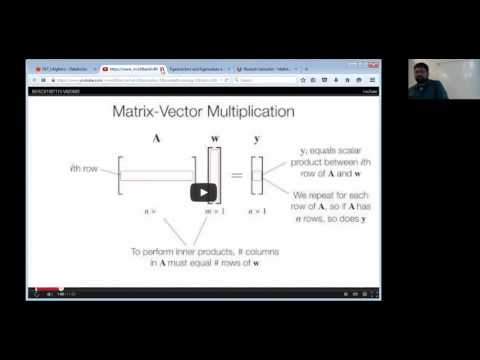// Databricks notebook source exported at Sat, 18 Jun 2016 23:27:13 UTC

# Scalable Data Science

### prepared by Raazesh Sainudiin and Sivanand Sivaram

The html source url of this databricks notebook and its recorded Uji:Let us visit an interactive visual cognitive tool for the basics ideas in linear regression:

The following video is a very concise and thorough treatment of linear regression for those who have taken the 200-level linear algebra. Others can fully understand it with some effort and revisiting.

#### Linear Regression by Ameet Talwalkar in BerkeleyX: CS190.1x Scalable Machine Learning

(watch now 11:13):Ridge regression has a Bayesian interpretation where the weights have a zero-mean Gaussian prior. See 7.5 in Murphy’s Machine Learning: A Probabilistic Perspective for details.

Please take notes in mark-down if you want.

For latex math within markdown you can do the following for in-line maths: $$\mathbf{A}_{i,j} \in \mathbb{R}^1$$. And to write maths in display mode do the following:

$\mathbf{A} \in \mathbb{R}^{m \times d}$

You will need to write such notes for your final project presentation!

#### MillonSongs Ridge Regression by Ameet Talwalkar in BerkeleyX: CS190.1x Scalable Machine Learning

(watch later 7:47):Covers the training, test and validation and grid search… ridger regression…

Take your own notes if you like.

#### Gradient Descent by Ameet Talwalkar in BerkeleyX: CS190.1x Scalable Machine Learning

(watch now 11:19):Please take notes if you want to.

Updated: